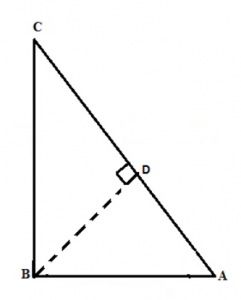# Explain the concept of Pythagorean Theorem

In a right-angled triangle, there are three sides: hypotenuse, perpendicular as well as the base. The base, as well as the perpendicular, make an angle of 90 degrees with each other. So, according to a Pythagorean theorem or Pythagoras theorem, “the square of the hypotenuse is equal to the sum of a base square and perpendicular square”. It is expressed as:

Hypotenuse2 = Base2 + Perpendicular2

This theorem is applicable for right-angled triangle only, although we will see its huge applications in trigonometry. Let us see the proof of this theorem.

Proof: Suppose a triangle ABC, right-angled at B.To Prove: AC2 = AB2 + BC2

Explanation: Draw a perpendicular BD to meet the side AC at D.

Since we know by the theorem: “If a perpendicular is drawn from the vertex of the right angle of a right triangle to the hypotenuse then triangles on both sides of the perpendicular are similar to the whole triangle and to each other”.

Therefore,

Hence,

AD/AB = AB/AC (Condition for similarity)

Or, AB2 = AD × AC ………………….(1)

Also, △BDC ~△ABC (Applying same theorem)

Therefore,

CD/BC = BC/AC (Condition for similarity)

Or,

BC2 = CD × AC ……………………..(2)

Adding the equations (1) and (2) we get,

AB2 + BC2 = AD × AC + CD × AC

AB2 + BC2 = AC (AD + CD)

Since, AD + CD = AC

Therefore, AC2 = AB2 + BC2

Hence, the Pythagorean theorem is proved.(1)(0)